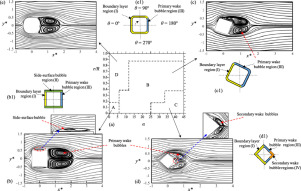International Journal of Mechanical Sciences ( IF 4.134 ) Pub Date : 2020-02-29 , DOI: 10.1016/j.ijmecsci.2020.105566
Mahbub Alam; Talaat Abdelhamid; Ahmad SohankarThe flow topology, heat transfer, and forces are numerically studied for a cylinder with its cross-section varied from square to circular by changing the cylinder corner radius ratio r/R = 0 – 1.0, where r is the corner radius and R is the half side width of the cylinder. The flow attack angle α is varied from 0° to 45°. The dependence of the flow on r/R and α leads to four flow patterns (A, B, C, and D). The effect of α on heat transfer and forces is large when r/R is small. The r/R largely affects the heat transfer at α < 30° and forces at α > 10°. The heat transfer for a given r/R enhances with increasing α. It is minimum at α = 0°, r/R = 0 and maximum at α = 45°, r/R = 0.5, with the maximum being 37% more than the minimum. The sensitiveness of heat transfer and forces to r/R and α is ascribed to the change in the boundary layer, side-surface bubble, primary wake bubble, and secondary wake bubble. The boundary layer largely contributes to the heat transfer while the primary wake bubble is largely connected to the forces. The vortex formation length is inversely linked to heat transfer and forces, with the heat transfer more influenced by formation length than by wake width.

down
wechat
bug